GATE  >  Agricultural Engineering- AG 2014 GATE Paper (Practice Test)

# Agricultural Engineering- AG 2014 GATE Paper (Practice Test)

Test Description

## 65 Questions MCQ Test GATE Past Year Papers for Practice (All Branches) | Agricultural Engineering- AG 2014 GATE Paper (Practice Test)

Agricultural Engineering- AG 2014 GATE Paper (Practice Test) for GATE 2023 is part of GATE Past Year Papers for Practice (All Branches) preparation. The Agricultural Engineering- AG 2014 GATE Paper (Practice Test) questions and answers have been prepared according to the GATE exam syllabus.The Agricultural Engineering- AG 2014 GATE Paper (Practice Test) MCQs are made for GATE 2023 Exam. Find important definitions, questions, notes, meanings, examples, exercises, MCQs and online tests for Agricultural Engineering- AG 2014 GATE Paper (Practice Test) below.
Solutions of Agricultural Engineering- AG 2014 GATE Paper (Practice Test) questions in English are available as part of our GATE Past Year Papers for Practice (All Branches) for GATE & Agricultural Engineering- AG 2014 GATE Paper (Practice Test) solutions in Hindi for GATE Past Year Papers for Practice (All Branches) course. Download more important topics, notes, lectures and mock test series for GATE Exam by signing up for free. Attempt Agricultural Engineering- AG 2014 GATE Paper (Practice Test) | 65 questions in 180 minutes | Mock test for GATE preparation | Free important questions MCQ to study GATE Past Year Papers for Practice (All Branches) for GATE Exam | Download free PDF with solutions
 1 Crore+ students have signed up on EduRev. Have you?
Agricultural Engineering- AG 2014 GATE Paper (Practice Test) - Question 1

### Q. 1 – Q. 5 carry one mark each. Choose the most appropriate word from the options given below to complete the following sentence. A person suffering from Alzheimer’s disease ______________short-term memory loss.

Agricultural Engineering- AG 2014 GATE Paper (Practice Test) - Question 2

### Choose the most appropriate word from the options given below to complete the following sentence. ____________ is the key to their happiness; they are satisfied with what they have.

Agricultural Engineering- AG 2014 GATE Paper (Practice Test) - Question 3

### Which of the following options is the closest in meaning to the sentence below? “As a woman, I have no country.”

*Answer can only contain numeric values
Agricultural Engineering- AG 2014 GATE Paper (Practice Test) - Question 4

In any given year, the probability of an earthquake greater than Magnitude 6 occurring in the Garhwal Himalayas is 0.04. The average time between successive occurrences of such earthquakes is ____ years

Agricultural Engineering- AG 2014 GATE Paper (Practice Test) - Question 5

The population of a new city is 5 million and is growing at 20% annually. How many years would it take to double at this growth rate?

Agricultural Engineering- AG 2014 GATE Paper (Practice Test) - Question 6

Q. 6 – Q. 10 carry two marks each.

In a group of four children, Som is younger to Riaz. Shiv is elder to Ansu. Ansu is youngest in the group. Which of the following statements is/are required to find the eldest child in the group? Statements

1. Shiv is younger to Riaz.
2. Shiv is elder to Som.

Agricultural Engineering- AG 2014 GATE Paper (Practice Test) - Question 7

Moving into a world of big data will require us to change our thinking about the merits of exactitude. To apply the conventional mindset of measurement to the digital, connected world of the twenty-first century is to miss a crucial point. As mentioned earlier, the obsession with exactness is an artefact of the information-deprived analog era. When data was sparse, every data point was critical, and thus great care was taken to avoid letting any point bias the analysis. From “BIG DATA” Viktor Mayer-Schonberger and Kenneth Cukier

The main point of the paragraph is:

Agricultural Engineering- AG 2014 GATE Paper (Practice Test) - Question 8

The total exports and revenues from the exports of a country are given in the two pie charts below. The pie chart for exports shows the quantity of each item as a percentage of the total quantity of exports. The pie chart for the revenues shows the percentage of the total revenue generated through export of each item. The total quantity of exports of all the items is 5 lakh tonnes and the total revenues are 250 crore rupees. What is the ratio of the revenue generated through export of Item 1 per kilogram to the revenue generated through export of Item for 4 kilogram?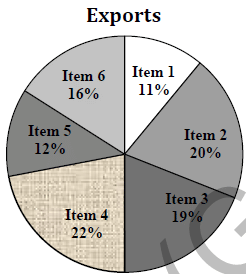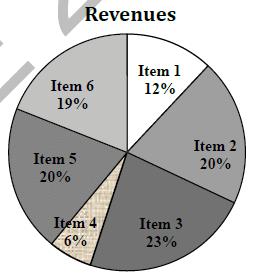Agricultural Engineering- AG 2014 GATE Paper (Practice Test) - Question 9

X is 1 km northeast of Y. Y is 1 km southeast of Z. W is 1 km west of Z. P is 1 km south of W. Q is 1 km east of P. What is the distance between X and Q in km?

*Answer can only contain numeric values
Agricultural Engineering- AG 2014 GATE Paper (Practice Test) - Question 10

10% of the population in a town is HIV+. A new diagnostic kit for HIV detection is available; this kit correctly identifies HIV+ individuals 95% of the time, and HIV individuals 89% of the time. A particular patient is tested using this kit and is found to be positive. The probability that the individual is actually positive is _______

Agricultural Engineering- AG 2014 GATE Paper (Practice Test) - Question 11

Q. 11– Q. 35 carry one mark each.

If two independent variables X and Y are uncorrelated then

Agricultural Engineering- AG 2014 GATE Paper (Practice Test) - Question 12

A partial differential equation containing dependent variable u is given by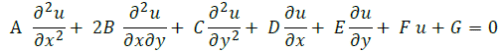where A, B, C, D, E, F and G are constants or functions of independent variables x or y only. AlsoG ≠ 0. The nature of the equation is

*Answer can only contain numeric values
Agricultural Engineering- AG 2014 GATE Paper (Practice Test) - Question 13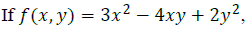the summation of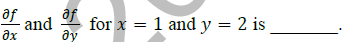Agricultural Engineering- AG 2014 GATE Paper (Practice Test) - Question 14

The brake power of a four-cylinder engine is 30 kW with all cylinders firing and 20 kW with any one cylinder cut. The mechanical efficiency of the engine in percent is

Agricultural Engineering- AG 2014 GATE Paper (Practice Test) - Question 15

A tractor is provided with an Ackerman steering gear mechanism. If b is the wheel base, c the distance between the front wheel pivot points, θ the steering angle of the inner wheel and φ the steering angle of the outer wheel, the fundamental relationship to be satisfied to avoid skidding of the two front wheels during a turn is given by

Agricultural Engineering- AG 2014 GATE Paper (Practice Test) - Question 16

Match the processes given in Group-I with the derived products given in Group-II.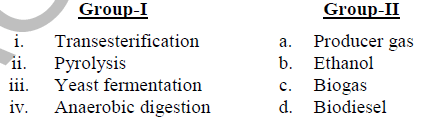Agricultural Engineering- AG 2014 GATE Paper (Practice Test) - Question 17

While operating a pedal thresher, 14 kg of paddy seed remained unthreshed for a throughput of 112 kg. If the quantity of the threshed seed obtained was 70 kg, the threshing efficiency in percent is

*Answer can only contain numeric values
Agricultural Engineering- AG 2014 GATE Paper (Practice Test) - Question 18

The pitch of the chain used in a chain drive motion is 38 mm. If the number of teeth on one of the sprockets is 35, the pitch circle diameter of the sprocket in m is_________.

*Answer can only contain numeric values
Agricultural Engineering- AG 2014 GATE Paper (Practice Test) - Question 19

A tractor tyre contains 31 L of air at a pressure of 190 kPa and a temperature of 30oC. Using R=8.314 J (gmol)-1 K-1 and molecular mass of air = 29 g (gmol)-1, the mass of air contained in the tyre is M × 10-3 kg. The value of M is________.

*Answer can only contain numeric values
Agricultural Engineering- AG 2014 GATE Paper (Practice Test) - Question 20

A flywheel and clutch assembly weighs 200 N and has a radius of gyration 150 mm. If the engine speed is 3000 rpm, the kinetic energy possessed by the rotating assembly in kJ is_______

*Answer can only contain numeric values
Agricultural Engineering- AG 2014 GATE Paper (Practice Test) - Question 21

A vertical conveyor reaper costing Rs. 75000 has a useful life of 8 years. Taking salvage value of the machine as 10% of the initial cost, the depreciated value after half of its useful life following straight line method will be Rs. ________.

Agricultural Engineering- AG 2014 GATE Paper (Practice Test) - Question 22

For a fully developed laminar flow through a smooth pipe, the relationship between friction factor (f) and Reynolds number (Re) is

Agricultural Engineering- AG 2014 GATE Paper (Practice Test) - Question 23

The process of determining the elevation of different points in a vertical plane is known as

Agricultural Engineering- AG 2014 GATE Paper (Practice Test) - Question 24

An imaginary surface obtained by joining the water levels in several observation wells driven in a confined aquifer is known as

Agricultural Engineering- AG 2014 GATE Paper (Practice Test) - Question 25

Several identical sprinkler nozzles, each having discharge Q (litre per minute), are spaced in a grid of size L (metre) × S (metre). The application rate in mm h-1 is

*Answer can only contain numeric values
Agricultural Engineering- AG 2014 GATE Paper (Practice Test) - Question 26

A 20 m chain used for surveying is found to be actually 19.7 m. If the actual distance is 1200 m, the chain distance in m will be________.

*Answer can only contain numeric values
Agricultural Engineering- AG 2014 GATE Paper (Practice Test) - Question 27

The brake power of a centrifugal pump having an impeller diameter of 200 mm is 1.86 kW. If the impeller is replaced with another impeller of 180 mm diameter, the brake power of the pump in kW will be _________.

*Answer can only contain numeric values
Agricultural Engineering- AG 2014 GATE Paper (Practice Test) - Question 28

The discharge of a single suction centrifugal pump operating against a total head of 12 m is 50 L s-1. If the pump is directly connected to a motor operating at 1440 rpm, the specific speed of the pump will be_______.

Agricultural Engineering- AG 2014 GATE Paper (Practice Test) - Question 29

A fat rich food product remains most stable in the water activity (aw) range of

Agricultural Engineering- AG 2014 GATE Paper (Practice Test) - Question 30

Identify the INCORRECT statement about the relevance of various dimensionless numbers in transport processes

Agricultural Engineering- AG 2014 GATE Paper (Practice Test) - Question 31

Select the most appropriate option about boiling and condensation processes as expressed by the statements P, Q and R.

P – The quantities of heat involved in evaporation and condensation of unit mass of fluid are identical
Q – The boiling and condensation of a single compound normally occur isothermally
R – The condensation is achieved at or below dew point and boiling occurs at triple point

Agricultural Engineering- AG 2014 GATE Paper (Practice Test) - Question 32

With increasing grain height in a deep cylindrical grain bin, the pressure at its base will

*Answer can only contain numeric values
Agricultural Engineering- AG 2014 GATE Paper (Practice Test) - Question 33

The observations recorded in a pulse de-husking operation are: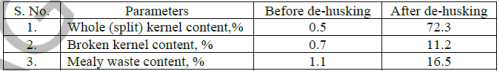For this operation the effectiveness of wholeness (in decimal) of kernels will be ________.

Agricultural Engineering- AG 2014 GATE Paper (Practice Test) - Question 34

Oil yield (Yo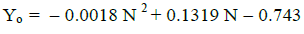For maximum oil yield, optimum stage of harvesting, in days after flowering is,

*Answer can only contain numeric values
Agricultural Engineering- AG 2014 GATE Paper (Practice Test) - Question 35

In a tray drying under adiabatic process, hot air enters the dryer at 50oC dry bulb temperature (DBT) and 10% relative humidity (RH) with enthalpy of 70.2 kJ per kg dry air. The air leaving the dryer gains 20% moisture. Considering saturated water vapour pressure as 12.349 kPa at 50oC DBT, the enthalpy of air leaving the dryer in kJ per kg dry air is __________.

Agricultural Engineering- AG 2014 GATE Paper (Practice Test) - Question 36

The eigenvalues of the matrix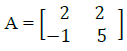are

Agricultural Engineering- AG 2014 GATE Paper (Practice Test) - Question 37

The value of line integral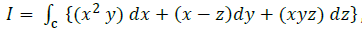where C is the arc of the
parabola y= x2 in the plane z = 2 from P (0, 0, 2) to Q (1, 1, 2), is

*Answer can only contain numeric values
Agricultural Engineering- AG 2014 GATE Paper (Practice Test) - Question 38

Consider the following set of linear equations
x1 + x2 + x3 = 6
2x1 + 2x2 + 3x3 = 14
3x1 + x2 + 2x3 = 14
The solution for this set exists only when the value of x2 is _________.

Agricultural Engineering- AG 2014 GATE Paper (Practice Test) - Question 39

If f(x) is a normal distribution with mean 8 and standard deviation 1, the value of f(x) for x= 10 is

Agricultural Engineering- AG 2014 GATE Paper (Practice Test) - Question 40

The value of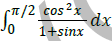is

Agricultural Engineering- AG 2014 GATE Paper (Practice Test) - Question 41

A four-stroke, four-cylinder diesel engine running at 2000 rpm develops brake power of 60 kW and the fuel consumption is 0.30 kg kW-1 h-1. The engine has a bore of 120 mm and stroke of 100 mm. If air-fuel ratio is 15:1 and air density is 1.15 kg m-3, the volumetric efficiency of the engine in percent in

Agricultural Engineering- AG 2014 GATE Paper (Practice Test) - Question 42

A four-wheel-drive tractor has a static weight of 50 kN with 40% weight on rear axle and 60% on front axle. The wheel base is 2 m. The tractor is pulling a disc harrow that exerts a level drawbar pull at a hitch height of 0.5 m from the ground. During the operation, when the dynamic reaction on each axle is same, the dynamic traction ratio developed by the tractor is

Agricultural Engineering- AG 2014 GATE Paper (Practice Test) - Question 43

A knapsack sprayer is provided with a nozzle having rated delivery of 0.5 L min-1 at a pressure of 270 kPa. For a pressure setting of 210 kPa, the application rate per unit orifice area is 0.24 L cm-2s-1. If the coefficient of discharge of the nozzle is 0.75, the diameter of the nozzle orifice in mm is

*Answer can only contain numeric values
Agricultural Engineering- AG 2014 GATE Paper (Practice Test) - Question 44

The day length (sunshine hours) on 31st May 2014 at a place in India (26o18’ N, 73o01’ E) will be ___________.

*Answer can only contain numeric values
Agricultural Engineering- AG 2014 GATE Paper (Practice Test) - Question 45

A tractor gear box has 8 forward speeds. The speed ratios (number of engine revolutions for one revolution of driving wheel) vary in exact geometrical progression. If the speed ratios in highest and lowest gears are 14.9 and 108.8, respectively, the geometric constant is ___________.

*Answer can only contain numeric values
Agricultural Engineering- AG 2014 GATE Paper (Practice Test) - Question 46

A multi-disc clutch has 4 steel discs and 3 bronze discs. The outside and inside diameters of contact surfaces are 250 mm and 180 mm, respectively. The coefficient of friction is 0.3 and axial force is 400 N. Assuming uniform wear, the power in kW that the clutch can transmit at 1000 rpm is _____.

Agricultural Engineering- AG 2014 GATE Paper (Practice Test) - Question 47

A cultivator with a working width of 1.2 m utilizes 95% of its width due to overlapping while operating at a forward speed of 2 km h-1. If the time lost in turning and other interruptions is 50 minutes per hectare, the field efficiency of the cultivator in percent is

*Answer can only contain numeric values
Agricultural Engineering- AG 2014 GATE Paper (Practice Test) - Question 48

A two-row horizontal plate potato planter with 0.6 m ridge spacing has 9 cups on each seed plate of 0.4 m diameter. For each revolution of the ground wheel, the seed plate makes half a revolution. The diameter of the ground wheel is 0.5 m. If the planter uses cut tubers each of 25 g mass, the seed rate in kg ha-1 is ____________.

Agricultural Engineering- AG 2014 GATE Paper (Practice Test) - Question 49

A hydraulic circuit uses a pump having a fixed displacement volume of 12.5 cm3 rev-1 driven at 1500 rpm. The pump has a volumetric efficiency of 85% and an overall efficiency of 75%. If the system pressure is set at 15 MPa by the relief valve, the power required to drive the pump in kW will be

*Answer can only contain numeric values
Agricultural Engineering- AG 2014 GATE Paper (Practice Test) - Question 50

A 200 m long horizontal pipe carries a discharge of 50 L s-1. The centre line of the pipe is 5 m above the datum. The diameter of the pipe tapers from 200 mm to 100 mm. Using g = 9.81 m s–2 and neglecting losses in the pipe, if the pressure at the larger end of the pipe is 100 kPa, the pressure at the other end of the pipe in kPa is __________.

*Answer can only contain numeric values
Agricultural Engineering- AG 2014 GATE Paper (Practice Test) - Question 51

A tile drainage system having 200 mm diameter lateral of 400 m length is used to drain an area with a drainage coefficient of 40 mm. Manning’s roughness coefficient for the drain pipe is 0.01. Drain pipes are laid at 0.3% slope. The spacing of the tile drain in m is __________.

Agricultural Engineering- AG 2014 GATE Paper (Practice Test) - Question 52

A crop has effective root zone depth of 1200 mm and monthly (30 days) crop evapotranspiration of 260 mm. The effective rainfall during 30 days period is 20 mm. The field capacity and permissible soil moisture depletion (volume basis) are 16% and 8%, respectively. The irrigation interval in days for the crop will be

Agricultural Engineering- AG 2014 GATE Paper (Practice Test) - Question 53

In a citrus orchard, planting is done at a spacing of 5 m × 5 m. The daily pan evaporation of the orchard is 6 mm. The pan coefficient, wetting factor (crop canopy factor) and crop coefficient are 0.8, 0.6 and 0.6, respectively. Four drippers each of 4 L h-1 discharge are used to irrigate each plant. The time of operation of drip irrigation system in hours will be

*Answer can only contain numeric values
Agricultural Engineering- AG 2014 GATE Paper (Practice Test) - Question 54

A 150 mm diameter well is driven fully in a confined aquifer of thickness 12 m. When pumping is done at a constant rate of 150 L min-1 for 10 h, the steady-state draw-downs are found to be 2.20 m and 0.02 m at distances of 14 m and 50 m, respectively from the centre of the well. The hydraulic conductivity of the aquifer in m day -1 is ___________.

Agricultural Engineering- AG 2014 GATE Paper (Practice Test) - Question 55

A triangular 5-hour unit hydrograph (UH1) of a catchment area of 400 km2 has a peak discharge of 60 m3 s-1. Another triangular 5-hour unit hydrograph (UH2) having the same base width as UH1 has a peak flow of 90 m3 s-1. The catchment area corresponding to UH2 in km2 is

*Answer can only contain numeric values
Agricultural Engineering- AG 2014 GATE Paper (Practice Test) - Question 56

A watershed has 1.8 km2 of cultivated area, 2.2 km2 of forest land and 1.4 km2 of grassed area. The runoff coefficient of cultivated area, forest land and grassed area are 0.25, 0.15 and 0.30, respectively. The main drainage channel has a fall of 25 m in the total length of 2.5 km. The Intensity-Duration-Frequency relationship for the watershed is expressed as,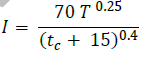where, I - intensity in cm h-1, T - recurrence interval in years and tc - time of concentration in minutes.
For a recurrence interval of 20 years, the peak rate of runoff for the watershed in m3s-1 will be __________.

Agricultural Engineering- AG 2014 GATE Paper (Practice Test) - Question 57

Bench terraces are to be constructed on a 15% hill slope. The batter slope is 1:1 and vertical interval is 2.5 m. The earth work in cutting is equal to filling. The quantum of earthwork in m3 ha–1 will be

*Answer can only contain numeric values
Agricultural Engineering- AG 2014 GATE Paper (Practice Test) - Question 58

A triangular shaped grassed waterway with a longitudinal slope of 2.5% is to carry a discharge of 1.5 m3 s-1 with a permissible velocity of 1.2 m s-1. The side slope of the channel is 1.5:1 (Horizontal:Vertical). Without considering free board, the top width of the channel in m is _________.

*Answer can only contain numeric values
Agricultural Engineering- AG 2014 GATE Paper (Practice Test) - Question 59

A container having volume 282.7 cm3 and total surface area 245 cm2 is completely filled with milk whose initial temperature is 25oC. The continually stirred milk container is suddenly exposed to a steam bath at 100oC. The overall heat transfer coefficient between steam and milk is 1136 W m-2 K-1. The properties of milk are: specific heat capacity = 3.9 kJ kg-1K-1, thermal conductivity = 0.54 W m-1K–1 and density = 1030 kg m-3. Neglecting thermal resistance and heat capacity of container walls, the necessary time required in seconds to heat milk up to the temperature of 85oC, will be ____________.

Agricultural Engineering- AG 2014 GATE Paper (Practice Test) - Question 60

In a continuous belt freezer, fish fillet at a feed rate of 1000 kg h–1 is frozen. The unfrozen fish having moisture content of 85% (wet basis) enters the freezer at 25oC and complete frozen fish exits at –20oC. Properties of fish are: latent heat of crystallization = 330 kJ kg–1, fixed freezing point = – 2.5oC, density = 1100 kg m-3, specific heat capacity above freezing point=3.60 kJ kg–1 K–1 and specific heat capacity below freezing point = 1.97 kJ kg–1 K–1. Neglecting other heat losses in the freezer, the power requirement of the compressor (in kW) having a coefficient of performance of 2.50 is,

*Answer can only contain numeric values
Agricultural Engineering- AG 2014 GATE Paper (Practice Test) - Question 61

A centrifuge with rotational speed of 1440 rpm is used for separating oil from a dispersion in which oil is present in the form of spherical globules of 47 μm diameter. The density of oil is 886 kg m-3. Separation occurs at an effective radius of 4 cm. Viscosity and density of water are 0.705 cP and 1000 kg m–3, respectively. The velocity of oil through water, in mm s-1, is ___________.

*Answer can only contain numeric values
Agricultural Engineering- AG 2014 GATE Paper (Practice Test) - Question 62

Energy required to grind a given mass of particles from a mean diameter of 12 mm to 4 mm is 12 kJ kg- 1. If energy consumed to grind the same mass of particles of 2 mm mean diameter to x mm mean diameter is 252 kJ kg-1, the value of x using Rittinger’s Law will be _______.

Agricultural Engineering- AG 2014 GATE Paper (Practice Test) - Question 63

A tray type paddy separator is used to separate paddy from a binary mixture of paddy and brown rice fed at the rate of 1600 kg h-1. Mass fractions of paddy in feed, separated paddy and separated rice are 0.2, 0.7 and 0.02, respectively. The mass of 1000 paddy grains is 24.5 g. Separation effectiveness and number of paddy grains recycled per second, respectively are

*Answer can only contain numeric values
Agricultural Engineering- AG 2014 GATE Paper (Practice Test) - Question 64

M kg of wheat at 9.8% moisture content (wet basis) is conditioned for 6 hours in 75 kg of water. A sample of 25 g conditioned wheat is crushed and dried in hot air oven at 130°C for 1 hour that yields 20.5 g of bone dried material. The value of M is _______.

*Answer can only contain numeric values
Agricultural Engineering- AG 2014 GATE Paper (Practice Test) - Question 65

In a food processing plant hot water at 90oC is needed at the rate of 140% of the commodity capacity. Steam at atmospheric pressure is used for heating the water available at 25oC. Latent heat of condensation at atmospheric pressure is 2257 kJ kg-1, net calorific value of hull is 12552 kJ kg-1 and specific heat capacity of water is 4.184 kJ kg-1 K-1. Steam is generated by utilizing the hulls received as by-product from the same plant at the rate of 22% of the commodity capacity. Assuming 20% heat loss, percentage of hull used with respect to the total available amount of hull is _____________.

## GATE Past Year Papers for Practice (All Branches)

407 docs|127 tests
Information about Agricultural Engineering- AG 2014 GATE Paper (Practice Test) Page
In this test you can find the Exam questions for Agricultural Engineering- AG 2014 GATE Paper (Practice Test) solved & explained in the simplest way possible. Besides giving Questions and answers for Agricultural Engineering- AG 2014 GATE Paper (Practice Test), EduRev gives you an ample number of Online tests for practice

## GATE Past Year Papers for Practice (All Branches)

407 docs|127 tests(Scan QR code)